Courses

# Computation of ΔH and ΔS for a Gas using Generalized Departure Functions Civil Engineering (CE) Notes | EduRev

## Civil Engineering (CE) : Computation of ΔH and ΔS for a Gas using Generalized Departure Functions Civil Engineering (CE) Notes | EduRev

The document Computation of ΔH and ΔS for a Gas using Generalized Departure Functions Civil Engineering (CE) Notes | EduRev is a part of the Civil Engineering (CE) Course Thermodynamics.
All you need of Civil Engineering (CE) at this link: Civil Engineering (CE)

Computation of ΔH and ΔS for a Gas using Generalized Departure Functions

The residual function equations presented in the last section are particularly useful for estimating finite changes in enthalpy and entropy for real gases undergoing change in either closed or open system processes. We consider that a pure fluid changes state from (T1 ,P1 ) to (T2 , P2 ); shown schematically in fig. 5.4.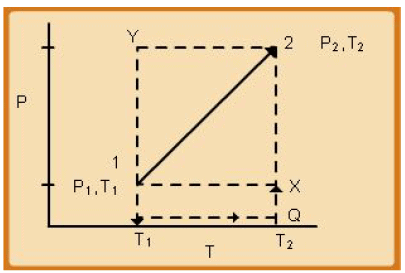Fig. 5.4 Schematic of a General Thermodynamic Process on P – T co-ordinates

Since the departure functions H R andS R capture deviations from ideal gas behaviour at the same temperature as the real gas, one can conceive of the pathway between states ‘1’ and ‘2’ to be decomposed into following steps (see fig 5.5):

a) Real gas state at (T1 ,P1 ) to ideal gas state (ig) at (T1 ,P1 )
b) Ideal gas state at (T1 ,P1 ) to ideal gas state at (T2,P2 )
c) Ideal gas state at (T2,P2 ) to real gas state at (T2,P2 )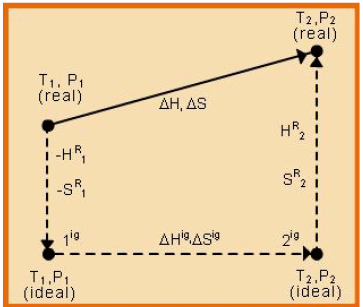5.5 Pathway for calculating ΔH and ΔS for Real Gases

• For step ‘a’ the change of enthalpy is given by: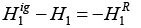• For step ‘b’ the change of enthalpy is given by: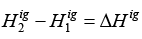• For step ‘c’ the change of enthalpy is given by: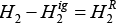Therefore, the overall change of enthalpy is given by: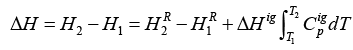Using eqn. 3.8: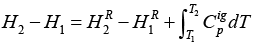......(5.63)

The same considerations apply for computing the change of entropy between the two states: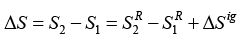Using eqn. 4.21: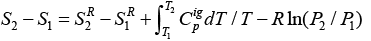......(5.64)

Generalized residual property relations may be used for calculation of change in internal energy i.e. U 2 −U1 for a process in the following manner:

U2 −U= (H2 − P2V2 ) −(H1 − P1V)

Or: U2 −U= (H2 −H1 ) −( P2V2 − P1V1 )                  ......(5.65)

The term (H2 −H1) can be calculated using eqn. 5.63, while the term ( P2V2 − P1V1 )

may be computed after obtaining Vand V2 applying the generalized compressibility factor approach. One may, however, also use the generalized residual property charts for internal energy for the same purpose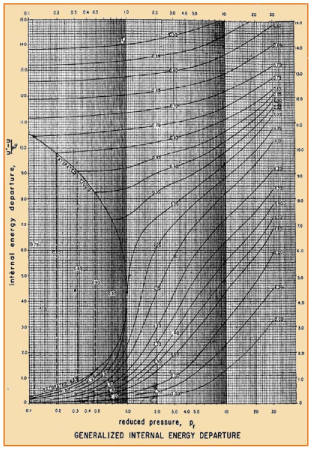Figure 5.6 Generalized internal energy departure functions using corresponding states

Offer running on EduRev: Apply code STAYHOME200 to get INR 200 off on our premium plan EduRev Infinity!

## Thermodynamics

56 videos|92 docs|33 tests

,

,

,

,

,

,

,

,

,

,

,

,

,

,

,

,

,

,

,

,

,

;